Physics
Fox

### Summary

• A series circuit is when the current goes along a single path. It looks like a simple loop: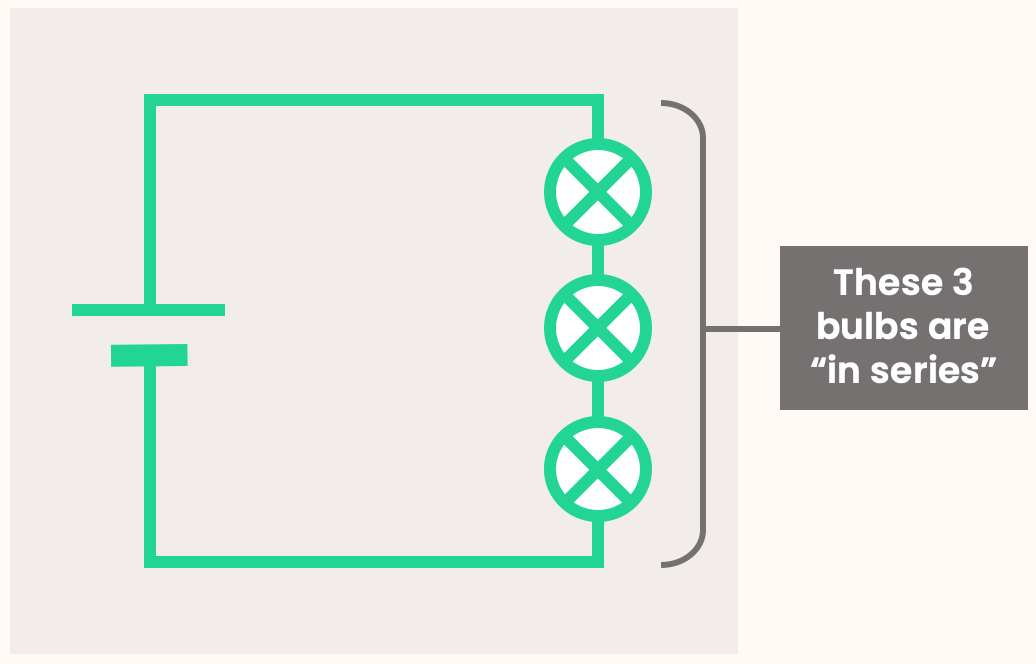• All components along the same path are said to be “in series”.

• In a series circuit, the current through each component is the same.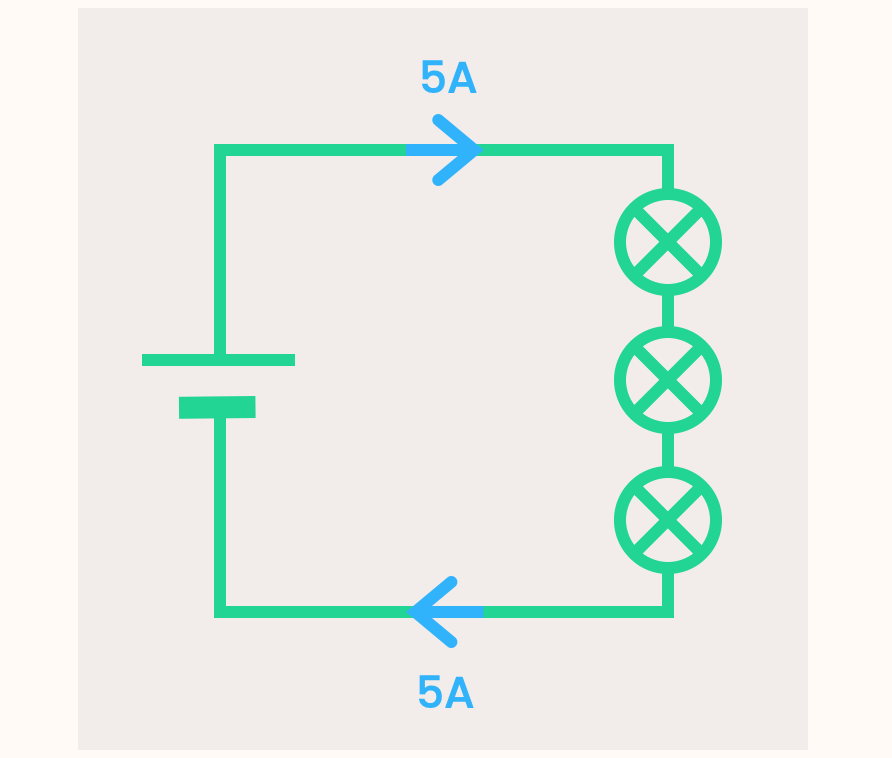• In a series circuit, the potential difference of the battery is shared between the components. Therefore, the potential difference across each component adds up to the potential difference of the battery. The p.d. might not be shared equally!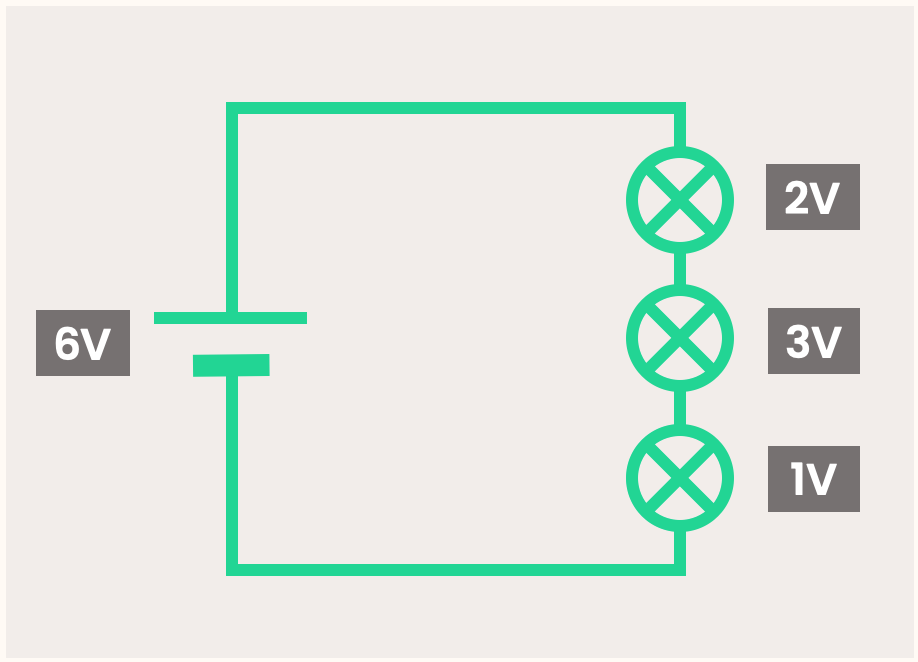• When multiple batteries/cells are in series, the potential difference adds together.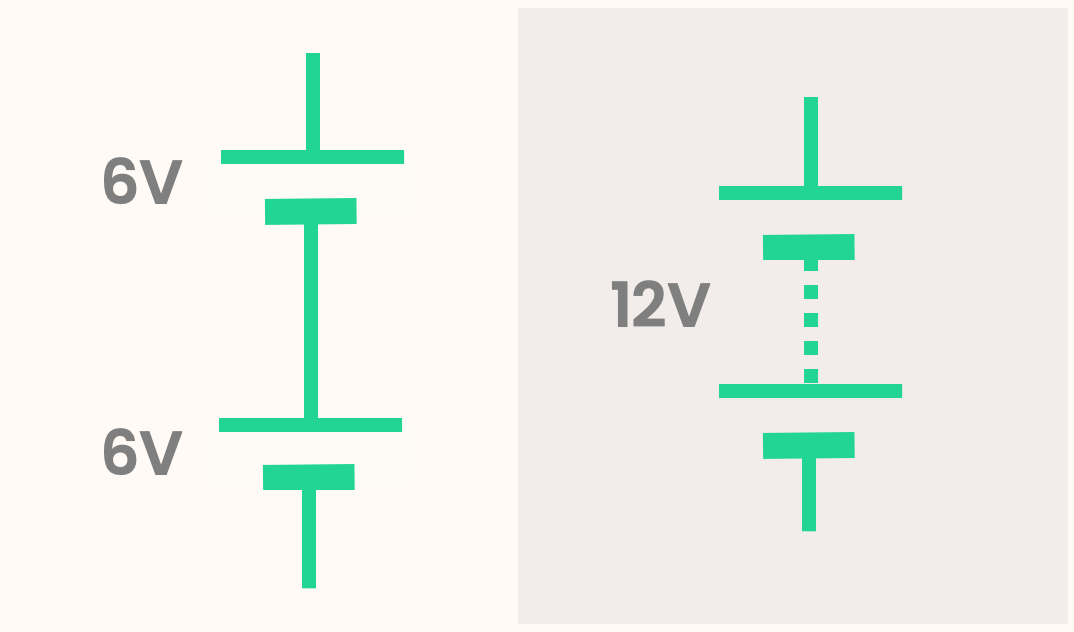Two 6V cells in series is the same as one 12V battery.

Once again, let's look at our water circuit: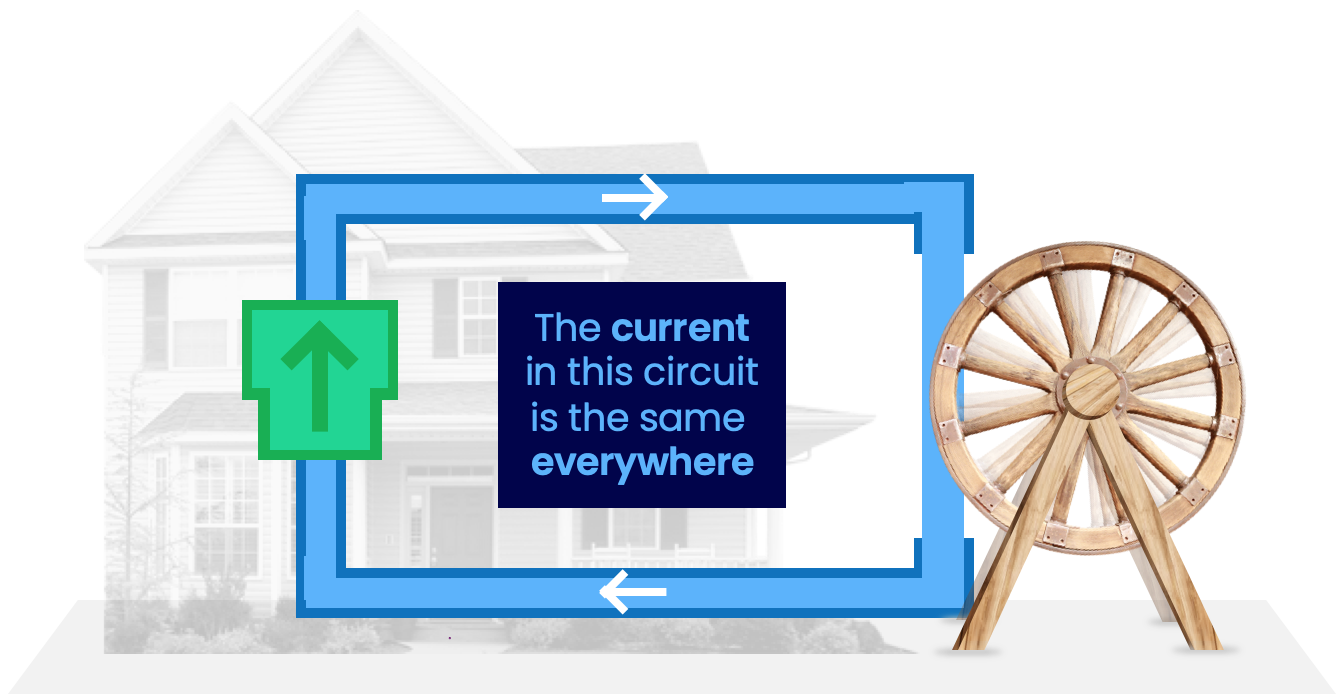When the circuit is just a simple loop like this, it is called a series circuit.

Note how the current anywhere in a series circuit is the same. This is because the current is flowing along a single path — it has nowhere else to go! So the current has to be the same throughout.

The pump takes the water to a higher potential energy. This energy is released when it comes back down on the right, and is used to power the waterwheel.

But what if we have multiple waterwheels?This energy would need to be shared between each waterwheel.

As always, what is true for our water circuit is also true for our electrical circuit. Let's look at an electrical series circuit: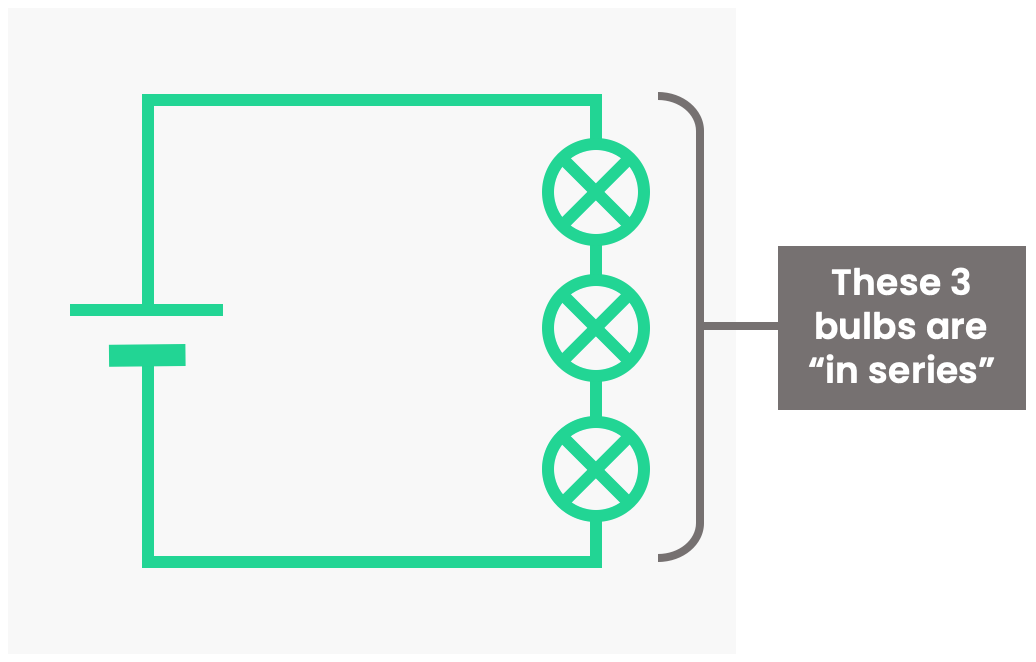Once again, it is a series circuit because it is a simple loop. A better definition of a series circuit is that the current only goes along one path. All components along the same path are said to be “in series”. A way to remember this: In many TV series, the episodes happen one after another, i.e. they follow one path.

As for the water circuit, the current everywhere in a series circuit is the same: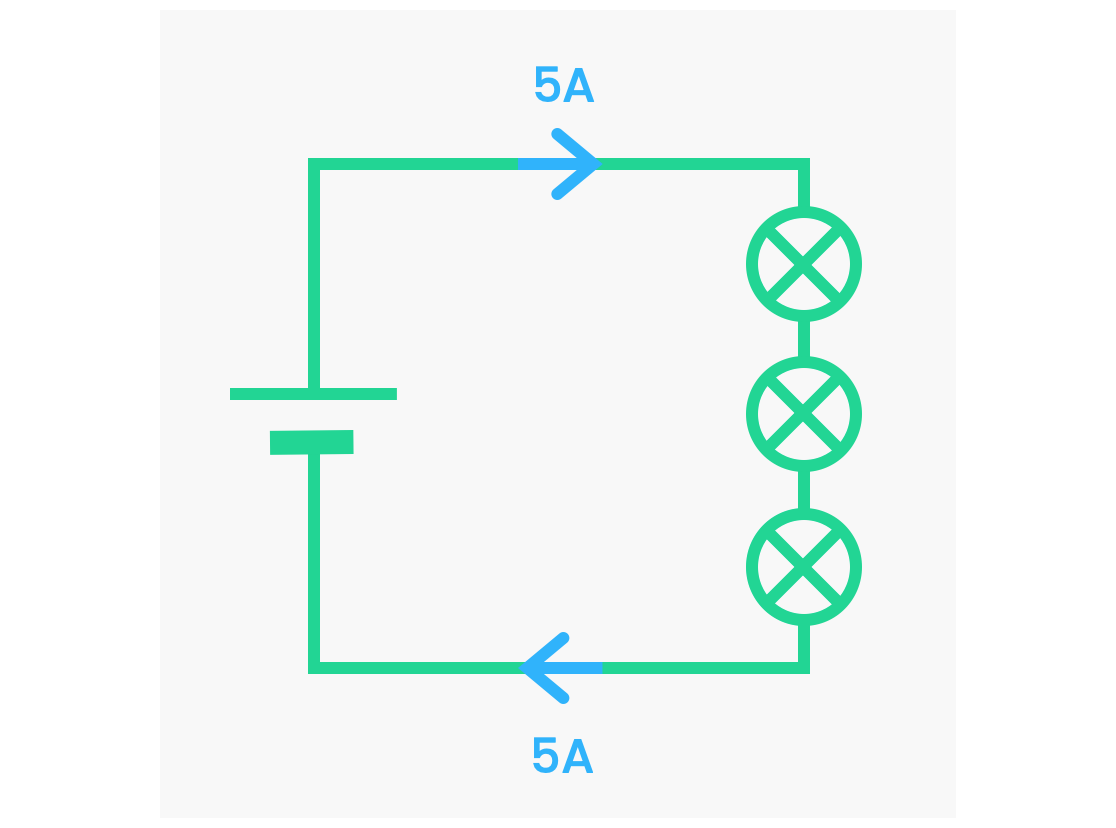The battery takes the electrons to a higher potential energy. This energy is released when the electrons come back down on the right, and is used to power the bulbs. The voltage of the battery is shared between the bulbs.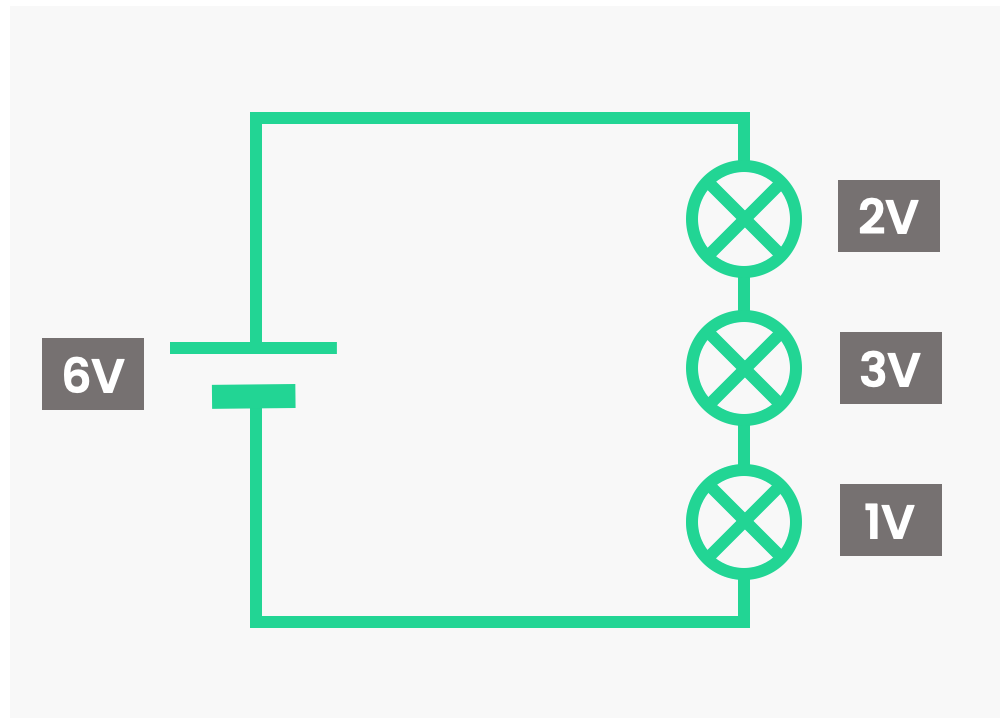Therefore, the voltage across each component adds up to the voltage of the battery. Note that the voltage might not be shared equally between the components. In the example above, One bulb has a p.d. of 2V, one has 3V, and another only 1V.

Why are they different? This is due to a property of the bulbs called their resistance. We'll cover resistance another time.

When batteries (or cells) are placed in series, the voltage of each battery/cell adds together. You do this to power more intense devices.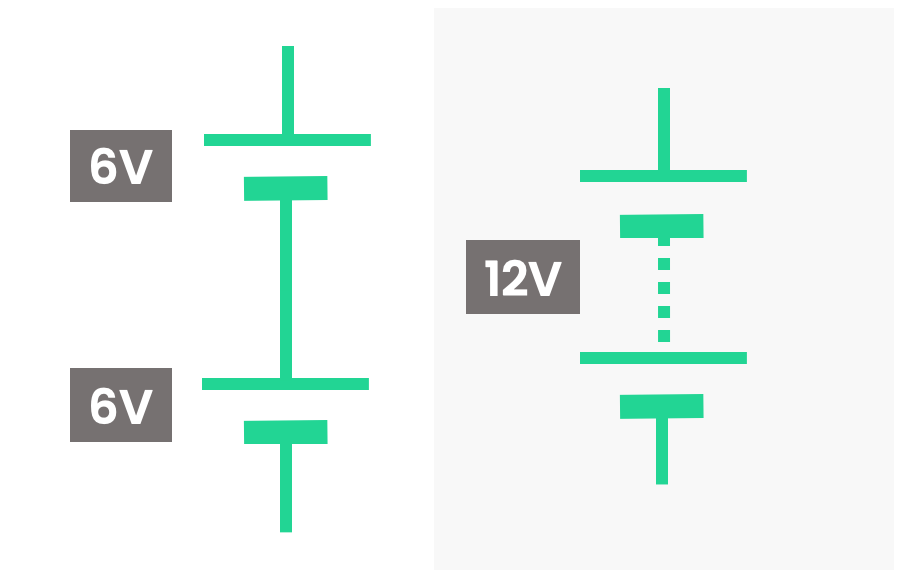Two 6V cells in series is the same as one 12V battery.

For example: In an electric toothbrush, you put two AA batteries in series, as the toothbrush would be too weak with just one AA battery. Each AA battery has a voltage of 1.5V, so when you put them in series it acts like a 3V battery: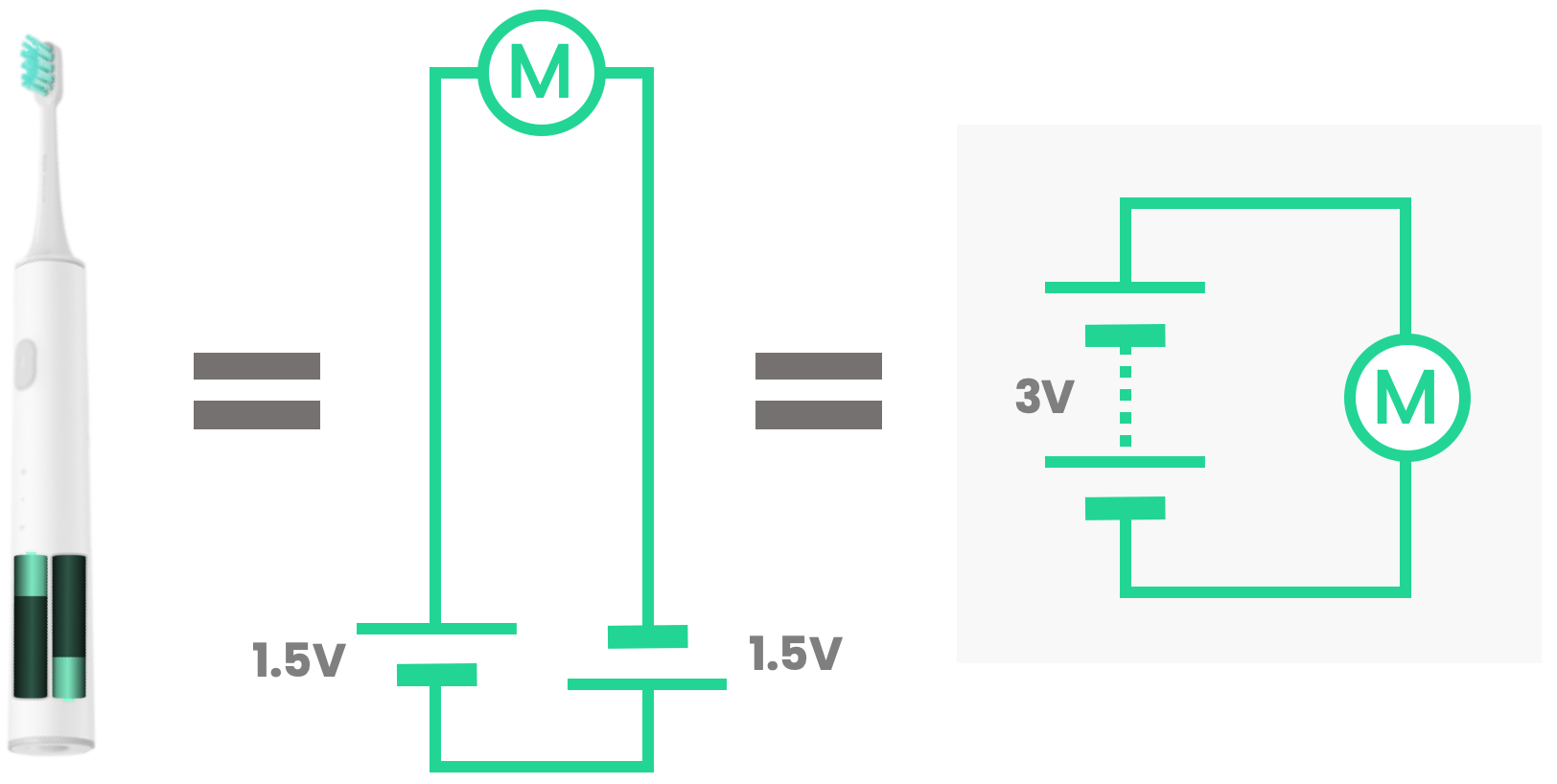(Ⓜ is the symbol for an electric motor)

The direction of the cells matters. If you put one in the opposite direction, the total potential difference is 1.5V - 1.5V = 0V. It's as if the batteries aren't even there! This is why you need both batteries to point the correct way.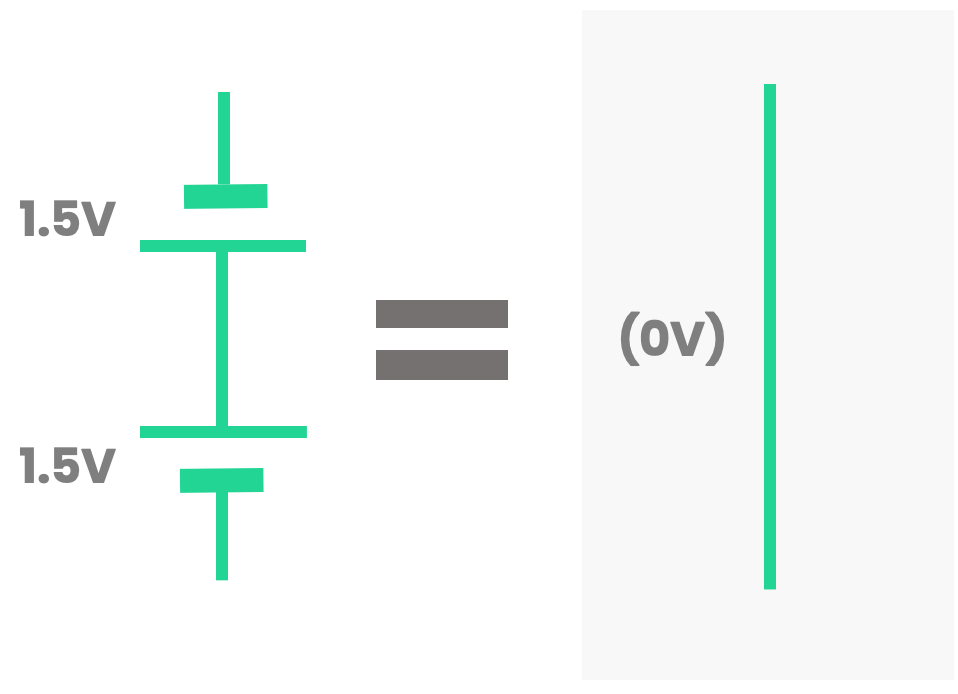When you have two (or more) cells in series, it is called a battery.

Quiz

### Congratulations!

12 of 12 questions completed

+ ⭐️ collected.

• You can change your display name at any time.
• at least 1 letter
• at least 1 number
• at least 8 characters